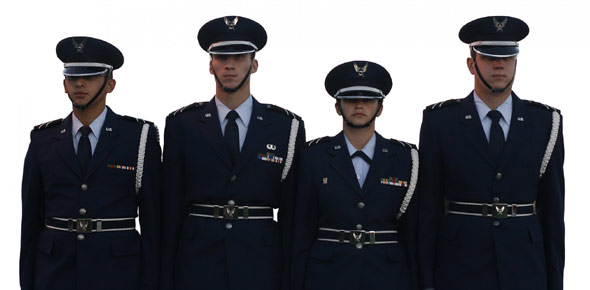# 3D1X3 CDC Volume 2 Ure

100 Questions | Attempts: 1484SettingsThe second one lol.

• 1.
(201)  Equipment used for generating, amplifying, and transmitting RF carrier is collectively called
• A.

• B.

An antenna.

• C.

A transmitter.

• D.

A transmission line.

• 2.
(201)  What basic receiver function involves having the transmitted electromagnetic wave pass through the receive antenna in such a manner as to induce a voltage in the antenna?
• A.

Reproduction.

• B.

Reception.

• C.

Detection.

• D.

Selection.

• 3.
(201)  The ability to reproduce the input signal accurately is the characteristics of the receiver's
• A.

Fidelity.

• B.

Capacity.

• C.

Sensitivity.

• D.

Selectivity.

• 4.
(202)  A combination of a transmitter and receiver that is built as a single unit and sharing common tuned circuits is called a
• A.

Transmitter.

• B.

Transceiver.

• C.

• D.

Coupler.

• 5.
(202)  Which of the following cannot be performed by the transceiver's computerized components and micro-circuitry?
• A.

Specific equipment faults.

• B.

Internal equipment tests.

• C.

Internal calibrations.

• D.

Repair itself.

• 6.
(203)  A transmission line that consists of a center conductor placed inside a metal tube functioning as the outer tube is called a
• A.

Flexible coaxial cable.

• B.

Rigid coaxial cable.

• C.

Waveguide.

• D.

• 7.
(203)  Which selection best describes the term "cutoff frequency" when discussing transmission line properties?
• A.

The frequency where XL becomes to low that the signal is shunted.

• B.

The frequency where XC causes the signal to be shunted.

• C.

The lowest frequency that the line can pass successfully.

• D.

The frequency at which standing waves are maximum.

• 8.
(203)  Using figure 1-10, determine the wavelength if the frequency is changed from 150 MHz to 250 MHz.
• A.

12.0 meters.

• B.

3.0 meters.

• C.

1.5 meters.

• D.

1.2 meters.

• 9.
(203)  Using figure 1-10, determine the electrical length if the frequency is changed from 150 MHz to 250 MHz.
• A.

8.33 meters.

• B.

8.33 wavelengths.

• C.

83.3 meters.

• D.

83.3 wavelengths.

• 10.
(203)  A nonresonant transmission line is a line
• A.

Having reflected waves.

• B.

Having no reflected waves.

• C.

With maximum voltage across its open termination.

• D.

With maximum voltages across its shorted termination.

• 11.
(203)  If a transmission line is terminated in a short
• A.

Current is at maximum and voltage at minimum at the termination.

• B.

Current is at minimum and voltage at maximum at the termination.

• C.

There would be a nominal amount of signal gain.

• D.

The line would be nonresonant.

• 12.
(204)  In a basic communication system, what converts radio frequency (RF) energy's current oscillation into electric and magnetic fields of force?
• A.

Antenna.

• B.

Coupler.

• C.

Transmitter.

• D.

Transmission lines.

• 13.
(204)  The concept that alternation current (AC) changes in magnitude and reverses its direction during each cycle is
• A.

An unproven hypothesis.

• B.

The definition of propagation.

• C.

• D.

What led to the discovery of direct current.

• 14.
(205)  If the electric field component travels in a plane perpendicular to the Earth's surface, the radio wave is
• A.

Magnetically polarized.

• B.

Horizontally polarized.

• C.

Circularly polarized.

• D.

Vertically polarized.

• 15.
(205)  What types of polarization do satellite terminals transmit and receive?
• A.

Transmit horizontal and receive vertical polarizations.

• B.

Transmit vertical and receive horizontal polarizations.

• C.

Transmit right hand and receive left hand circular polarizations.

• D.

Transmit left hand and receive right hand circular polarizations.

• 16.
(206)  What measurement is used to determine whether an antenna is resonant at a particular frequency?
• A.

Distortion.

• B.

Standing wave ratio.

• C.

Signal-to-noise ratio.

• D.

Percent of modulation.

• 17.
(206)  The ability of an antenna to both receive and transmit equally well is known as the antenna's
• A.

Bandwidth.

• B.

Resonance.

• C.

Reciprocity.

• D.

Effectiveness.

• 18.
(206)  The standard used to measure the radiating effectiveness (gain) of an antenna system is the
• A.

Marconi antenna.

• B.

Isotropic antenna.

• C.

Hertz antenna.

• D.

Whip antenna.

• 19.
(206)  What does the effectiveness of an entire transmitting and receiving system depend largely upon?
• A.

Impedance matching.

• B.

Antenna distance.

• C.

Antenna properties.

• D.

Line characteristics.

• 20.
• A.

Omnidirectional.

• B.

Unidirectional.

• C.

Bidirectional.

• D.

Directional.

• 21.
(207)  Which antenna type is usually used on long-range, point-to-point circuits where the concentrated radio energy is needed for circuitry reliability?
• A.

Omnidirectional.

• B.

Unidirectional.

• C.

Bidirectional.

• D.

Circular.

• 22.
(208)  Ungrounded lengths of wire specifically designed to be either a half-wavelength or more than a full wavelength long is called a
• A.

Reflector.

• B.

Hertz antenna.

• C.

Marconi antenna.

• D.

Vertical monopole.

• 23.
(209)  A long-wire's takeoff angle depends on the antenna's
• A.

Length.

• B.

Directivity.

• C.

Front-to-back ratio.

• D.

Standing wave ratio.

• 24.
(209)  All antennas used in satellite communications are designed to be
• A.

Circular directional.

• B.

Omnidirectional.

• C.

Bidirectional.

• D.

Directional.

• 25.
(209)  A commmon satellite antenna is the
• A.

Whip.

• B.

Discone.

• C.

Parabolic.

• D.

Long-wire.

## Related TopicsBack to top
×

Wait!
Here's an interesting quiz for you.